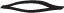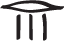# Year 7

## Number and Algebra

### Expressing one quantity as a fraction of a second

In general, the ancient civilisations avoided fractions by giving special names to parts of various measures. We still do this today. For example, instead of saying $$1\dfrac{13}{60}$$ hours, we say 1 hour 13 minutes.

Egyptian fraction notation was developed in the Middle Kingdom of Egypt (2080−1640 BC).With few exceptions, they wrote all their fractions as unit fractions, that is, with numerator 1. To write the unit fractions used in their fraction notation, the Egyptians placed the hieroglyphabove the numeral for the denominator. For example:

$$\dfrac{1}{3}=$$Traditionally, the term 'fraction' was used to describe a part of a whole. The word comes from the Latin word fractus, meaning 'broken into pieces'. In this module, we will take a fraction to mean a non-negative rational number, that is, a number of the form $$\dfrac{m}{n}$$, where n is a positive integer and m is a positive integer or 0.

Fractions arise naturally in everyday situations involving sharing, cutting up and proportions. For example, the bathtub was one-third full; three-quarters of the class walk to school; the time is a quarter-past 10 in the morning; the score at half-time was 106 to 98 points. Fractions between 0 and 1 describe parts of a whole. Fractions extend the whole numbers to a number system in which division by a non-zero number always makes sense.